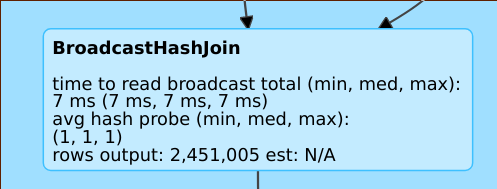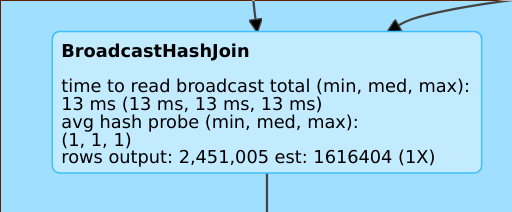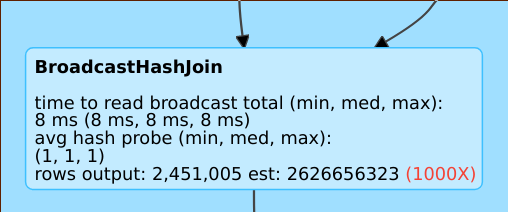# Cost-based optimizer

Spark SQL can use a cost-based optimizer (CBO) to improve query plans. This is especially useful for queries with multiple joins. For this to work it is critical to collect table and column statistics and keep them up to date.

## Collect statistics

To get the full benefit of the CBO it is important to collect both column statistics and table statistics. Statistics can be collected using the Analyze Table command.

Tip

To maintain the statistics up-to-date, run `ANALYZE TABLE` after writing to the table.

## Verify query plans

There are several ways to verify the query plan.

### `EXPLAIN` command

To check if the plan uses statistics, use the SQL commands

• Databricks Runtime 7.x and above: EXPLAIN

• Databricks Runtime 5.5 LTS and 6.x: Explain

If statistics are missing then the query plan might not be optimal.

```== Optimized Logical Plan ==
Aggregate [s_store_sk], [s_store_sk, count(1) AS count(1)L], Statistics(sizeInBytes=20.0 B, rowCount=1, hints=none)
+- Project [s_store_sk], Statistics(sizeInBytes=18.5 MB, rowCount=1.62E+6, hints=none)
+- Join Inner, (d_date_sk = ss_sold_date_sk), Statistics(sizeInBytes=30.8 MB, rowCount=1.62E+6, hints=none)
:- Project [ss_sold_date_sk, s_store_sk], Statistics(sizeInBytes=39.1 GB, rowCount=2.63E+9, hints=none)
:  +- Join Inner, (s_store_sk = ss_store_sk), Statistics(sizeInBytes=48.9 GB, rowCount=2.63E+9, hints=none)
:     :- Project [ss_store_sk, ss_sold_date_sk], Statistics(sizeInBytes=39.1 GB, rowCount=2.63E+9, hints=none)
:     :  +- Filter (isnotnull(ss_store_sk) && isnotnull(ss_sold_date_sk)), Statistics(sizeInBytes=39.1 GB, rowCount=2.63E+9, hints=none)
:     :     +- Relation[ss_store_sk,ss_sold_date_sk] parquet, Statistics(sizeInBytes=134.6 GB, rowCount=2.88E+9, hints=none)
:     +- Project [s_store_sk], Statistics(sizeInBytes=11.7 KB, rowCount=1.00E+3, hints=none)
:        +- Filter isnotnull(s_store_sk), Statistics(sizeInBytes=11.7 KB, rowCount=1.00E+3, hints=none)
:           +- Relation[s_store_sk] parquet, Statistics(sizeInBytes=88.0 KB, rowCount=1.00E+3, hints=none)
+- Project [d_date_sk], Statistics(sizeInBytes=12.0 B, rowCount=1, hints=none)
+- Filter ((((isnotnull(d_year) && isnotnull(d_date)) && (d_year = 2000)) && (d_date = 2000-12-31)) && isnotnull(d_date_sk)), Statistics(sizeInBytes=38.0 B, rowCount=1, hints=none)
+- Relation[d_date_sk,d_date,d_year] parquet, Statistics(sizeInBytes=1786.7 KB, rowCount=7.30E+4, hints=none)
```

Important

The `rowCount` statistic is especially important for queries with multiple joins. If `rowCount` is missing, it means there is not enough information to calculate it (that is, some required columns do not have statistics).

### Spark SQL UI

Use the Spark SQL UI page to see the executed plan and accuracy of the statistics.Missing estimate

A line such as `rows output: 2,451,005 est: N/A` means that this operator produces approximately 2M rows and there were no statistics available.Good estimate

A line such as `rows output: 2,451,005 est: 1616404 (1X)` means that this operator produces approx. 2M rows, while the estimate was approx. 1.6M and the estimation error factor was 1.A line such as `rows output: 2,451,005 est: 2626656323` means that this operator produces approximately 2M rows while the estimate was 2B rows, so the estimation error factor was 1000.
The CBO is enabled by default. You disable the CBO by changing the `spark.sql.cbo.enabled` flag.
```spark.conf.set("spark.sql.cbo.enabled", false)